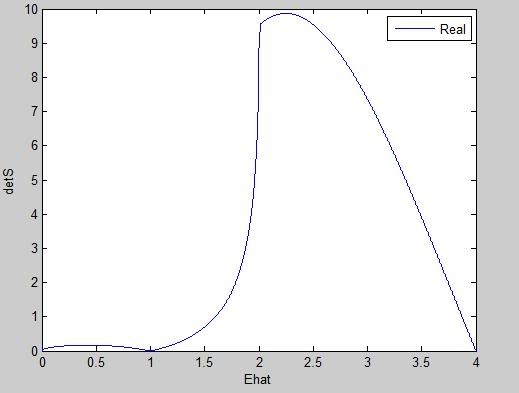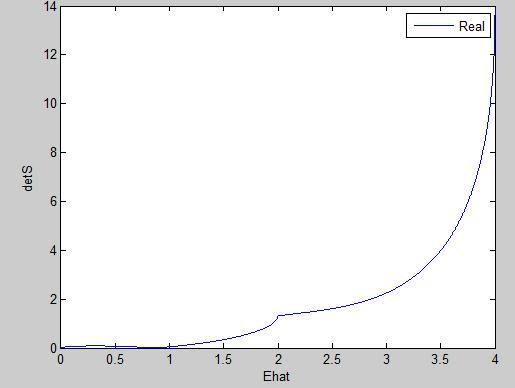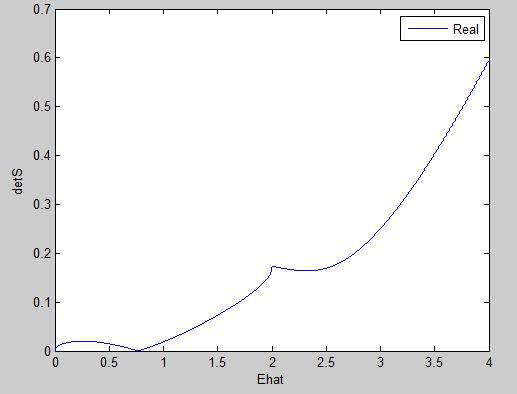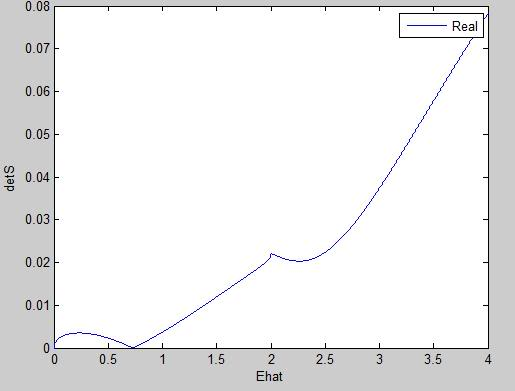# Do these solutions look vaguely correct?

## Homework StatementI wrote a whole heap of matlab code, and I have no idea whether my answers, or the plots of my answers are anywhere in the vicinity of looking right. I was hoping someone with a lil bit of experience in this type of physics would be able to tell me if I'm on the right track..

None.

## The Attempt at a Solution

for $$\alpha$$ = 1for $$\alpha$$ = 2for $$\alpha$$ = 5for $$\alpha$$ = 10## Answers and Replies

This is a variation of the finite square well.

As a result, the wavefuctions and energies should be solvable analytically (analytically meaning you can form closed form expressions for the variables involved), although the relationships for the boundary conditions may yield transcendental equations.

However, if you have to use matlab, you should bear in mind that it is similar to a finite square well, and the energies should be about the same order of magnitude.

I think the WKB approximation may be used here.

You may find this numerical solver useful:

http://qbx6.ltu.edu/s_schneider/physlets/main/finite_sqr_well.shtml [Broken]

What do the x-axis and y-axis represent?

Last edited by a moderator:
the x-axis is Ehat - the energy, normalised. So: Ehat = E $$\frac{L}{2}$$, where L is the length of gap in my "well"

and the y-axis is detS - the determinant of the matrix I created from the boundary and interface conditions. So each x-axis crossing should give me an energy eigenvalue shouldn't it?

if so, why am I only getting one zero-crossing in each graph?

Last edited:
Could I take a look at your S matrix?

yep.

S =

$$e^{-a} \ \ \ \ \ -e^{-jk} \ \ \ \ \ -e^{jk} \ \ \ \ \ 0$$
$$ae^{-a} \ \ \ \ \ -jke^{-jk} \ \ \ \ \ jke^{jk} \ \ \ \ \ 0$$
$$0 \ \ \ \ \ e^{jk} \ \ \ \ \ e^{-jk} \ \ \ \ \ -e^{-a}$$
$$0 \ \ \ \ \ jke^{jk} \ \ \ \ \ -jke^{-jk} \ \ \ \ \ ae^{-a}$$

This is the matrix "S" when either side of the potential well are the same height. This is copied from the teachers notes that he wrote on the board.

but when region 3 is heigher than region 1, as in the assignment question i got given..

i tried introducting a new vector, B. So that my matrix S changed thus:

$$e^{-a} \ \ \ \ \ -e^{-jk} \ \ \ \ \ -e^{jk} \ \ \ \ \ 0$$
$$ae^{-a} \ \ \ \ \ -jke^{-jk} \ \ \ \ \ jke^{jk} \ \ \ \ \ 0$$
$$0 \ \ \ \ \ e^{jk} \ \ \ \ \ e^{-jk} \ \ \ \ \ -e^{-B}$$
$$0 \ \ \ \ \ jke^{jk} \ \ \ \ \ -jke^{-jk} \ \ \ \ \ Be^{-B}$$

where a = $$\frac{pi}{2}\sqrt{Vo-E}$$
and B = $$\frac{pi}{2}\sqrt{\alpha Vo-E}$$

and I think this might be where I'm going wrong..

Your S-Matrix seems fine.

When you say real, is it |Re[function]| or Abs[function] (ie, Re[x+ iy] = x, Abs[x + iy] = $$\sqrt{x^2 + y^2}$$)?

I worked out a solution in Mathematica, using a slightly different method (basically equating all boundary conditions) and forming the equation

$$Tan(k L) = Something Horrible$$
$$k = \sqrt{{\frac{\hbar^2 E}{2m}}}$$

From what I observe, the 0 at the origin is a mathematical artifact that comes from dividing by 0.

$$Something Horrible = \frac{Something_a}{\frac{Something_b}{0} + Something_c}$$

Additionally, I found it necessary to choose a value for $$V_0$$ in the form:
$$z_0 = \sqrt{\frac{2 m V_0 L^2}{\hbar^2}}$$

Does the question indicate what $$V_0$$ should be, or am I mistaken?

There will be a minimum of one bound state. The maximum number of bound states (zero crossings) depends on how large $$V_0$$ is.

I think your plots are alright. The only thing to note is that in your first plot $$\alpha = 1$$, it appears that there are two crossings. If you reduce your scale, I think there's a chance that the line at 4 approaches the x axis closely,but doesn't actually touch it.

This would explain why that there's only one crossing for the other alphas.

Furthermore, depending on how you implemented the boundary conditions, the S matrix will not be valid beyond a certain energy for the excited states - that energy being $$\alpha V_o > E > V_o$$, which represent plane waves extending from -infinity and being reflected off the $$\alpha V_o$$ barrier, while $$E > \alpha V_o$$ would just give plane waves from +-infinity

Last edited:
Must I have a value for Vo to find the eigenenergy values?

In all the examples my teacher has ever given, he always seems to write Vo, but not actually specify what it is.

Must I have a value for Vo to find the eigenenergy values?

In all the examples my teacher has ever given, he always seems to write Vo, but not actually specify what it is.

Vo needs to be specified.

For example, in a finite square well, the number of bound states and their energies depends on Vo. As Vo increases, the number of bound states changes as does their energies (slightly).

When Vo -> infinity, we get the infinite square well which has an infinite number of bound states.

What do you mean by bound states?

I'm really sorry to be such a newbie.!

A bound state is a state with total energy Kinetic Energy + Potential Energy (appropriately scaled) = E + V < 0.

Alternatively, it is a state whose motion is restricted to a finite region, ie. bounded.

For example, for planets orbiting around the sun, the Kinetic Energy + Gravitational Potential Energy of the system < 0, so they represent bound states (A good thing for Earth!).

One point of note is that the absolute value of potential is arbitrary - only relative values matter. As such, we typically set Potential Energy to be 0 for points that are very far away, and work backwards to find Potential Energy at the origin or example.

So for gravitationally bound systems, the Potential Energy is set to be 0 at infinity, and we find out that it becomes negative for points not at infinity.

Similarly for this modified finite square well, we would set Vo = 0 at infinity, so the Potential Energy of a particle in the well is -Vo for the purposes of determining whether it is bound or not.

The reason we do not set $$\alpha V_o$$ = 0 at infinite instead is because a particle with energy = Vo would already be able to travel to infinity, let alone a particle with even more energy = $$\alpha V_o$$; ie. not bounded by the second definition.

I get the feeling that you are doing Quantum Mechanics without really understanding what is going on - I would encourage reading up on introductory texts such as http://www.amazon.com/dp/0131911759/?tag=pfamazon01-20, or the plethora of online lecture notes.

Last edited:
Quantum mechanics without really knowing what is going on - that is an accurate description. I'm studying electronic engineering - but we're all forced to take on this subject which is called Electronc Materials. I'm finding it tough with little to no background in Physics.

Anyway, I've bought the book you suggested on eBay. I'm going into uni tomorrow to see if I can recruit some help from somebody. I'll post whatever progress I've made with my assignment here tomorrow night - either as a JPEG or PDF, so you can see. You might find it amusing because I'm so bad.